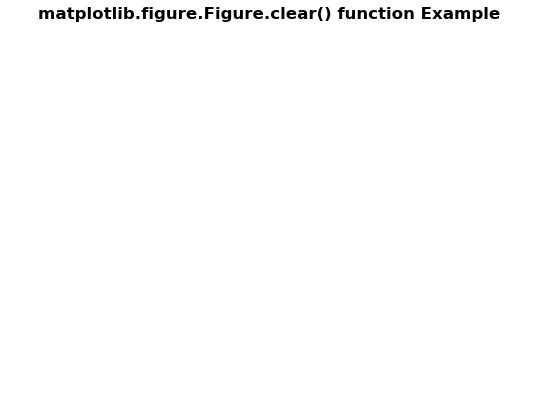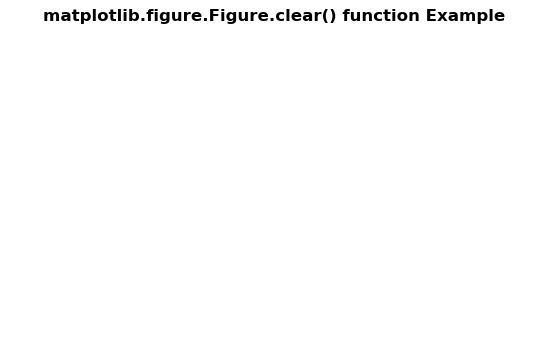# Matplotlib.figure.Figure.clear() in Python

Matplotlib is a library in Python and it is numerical – mathematical extension for NumPy library. The figure module provides the top-level Artist, the Figure, which contains all the plot elements. This module is used to control the default spacing of the subplots and top level container for all plot elements.

## matplotlib.figure.Figure.clear() function

The clear() method figure module of matplotlib library is used to Clear the figure.

Syntax: clear(self, keep_observers=False)

Parameters: This accept the following parameters that are described below:

• keep_observers: This parameter is the boolean value.

Returns: This method does not return any value.

Below examples illustrate the matplotlib.figure.Figure.clear() function in matplotlib.figure:

Example 1:

 `# Implementation of matplotlib function ` `import` `numpy as np ` `import` `matplotlib.pyplot as plt ` `     `  `fig, ax ``=` `plt.subplots() ` ` `  `ax.set_xlabel(``'x-axis'``) ` `ax.set_ylabel(``'y-axis'``) ` ` `  `ax.plot([``1``, ``2``, ``3``]) ` `ax.grid(``True``) ` ` `  `fig.clear(``True``) ` `  `  `fig.suptitle('matplotlib.figure.Figure.clear() \ ` `function Example\n\n', fontweight ``=``"bold"``) ` ` `  `plt.show() `

Output:Example 2:

 `# Implementation of matplotlib function ` `import` `numpy as np ` `import` `matplotlib.pyplot as plt ` `     `  `t ``=` `np.linspace(``0.0``, ``2.0``, ``201``) ` `s ``=` `np.sin(``2` `*` `np.pi ``*` `t) ` `  `  `fig, [ax, ax1] ``=` `plt.subplots(``2``, ``1``,  ` `                              ``sharex ``=` `True``) ` `  `  `ax.set_ylabel(``'y-axis'``) ` `ax.plot(t, s) ` `ax.grid(``True``) ` `ax.set_title(``'Sample Example'``, ` `             ``fontsize ``=` `12``, ` `             ``fontweight ``=``'bold'``) ` `  `  `ax1.set_ylabel(``'y-axis'``) ` `ax1.plot(t, s) ` `ax1.grid(``True``) ` ` `  `fig.clear(``False``) ` `  `  `fig.suptitle('matplotlib.figure.Figure.clear() \ ` `function Example\n\n', fontweight ``=``"bold"``) ` ` `  `plt.show() `

Output:Whether you're preparing for your first job interview or aiming to upskill in this ever-evolving tech landscape, GeeksforGeeks Courses are your key to success. We provide top-quality content at affordable prices, all geared towards accelerating your growth in a time-bound manner. Join the millions we've already empowered, and we're here to do the same for you. Don't miss out - check it out now!

Previous
Next